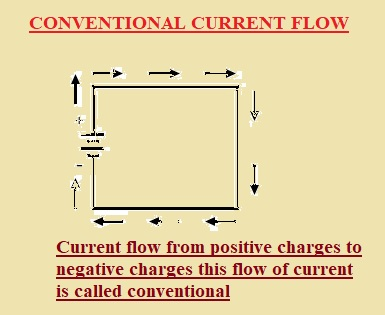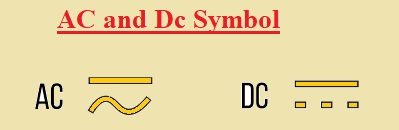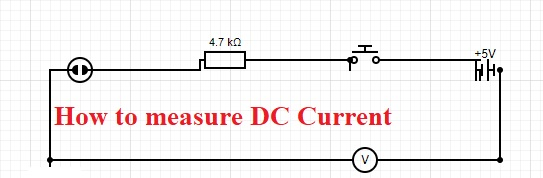Hello, readers welcome to the new post. Today we will learn WHAT IS DIRECT CURRENT? DC current or direct current was first time found by Alessandro Volta who was a physicist and he also invented the first chemical battery in 1799.  The complete description of electric current was understood through the theory of Andre-Marie Ampere. He said that current flows are due to positive charges. In this experiment, he discussed the current flow of high positive charges to lowes positive charges

In this post, we will discuss the different points of Direct current and finds its practical applications. So let’s get started WHAT IS DIRECT CURRENT?

### Introduction to DIRECT CURRENT?

• Direct current is caused due to unidirectional flow of electric charges.  The cell is an example of dc current.
• Dc current flows through conductive material like a wire which also flows from semiconductor materials insulators etc.
• Dc current is also called galvanic current. For AC to Dc conversion rectifiers are used that consist of diodes
• For dc to ac conversion inverter circuits are used.
• There are different applications of dc current like battery charging and power to motors.
• Dc current used in railway system high voltage dc current used to for high power transmission to distant areas### WHAT IS CONVENTIONAL CURRENT FLOW

• The current flow from positive charges to negative charges this flow of current is called conventional current flow. It is also called the flow of current from high positive charges to low positive charges. Normally flow of current is conventional flow.### What is ELECTRON CURRENT FLOW

• The flow of negative charges or electrons from a negative point to fewer electron parts is called conventional current flow

### HOW TO CALCULATE CURRENT

• The flow rate of charges is called current. It is also calculated by measuring the charge flow through certain points at specific time intervals. Its SI unit is Ampere
• The formula used for measuring the current is
• Q/t=I
• Here Q is charged t is time and I is current

### Differences between AC and DC

• AC is current that changes its polarity after some time interval and dc current has the same polarity. here some differences are discussed between AC and DC.
• Direct current does not change direction and AC current changes when flow in a circuit
• The frequency of AC current is fifty or sixty on the bases of the country and DC current has zero since its polarity, not changes
• In AC current electrons move back and forth while in DC electrons flow in a single direction
• The magnitude of AC varies for different time intervals and DC has the same magnitude
• AC has a 0 to 1 power factor and Dc has always one power factor
• Impedance is ac parameter and dc has resistance
• Wave for dc is pure dc or pulsating while AC has square, triangular, and sinusoidal
• Rectifier used for AC to dc conversion and DC to AC inverter used
• Ac can operate resistive inductive or capacitance load and dc drive only resistive loads
• AC current produces through ac generators like synchronous generators, and induction generators and DC current produces through a battery or DC generator### DC Current Symbol

• The dc current has a constant value so its symbol is a straight line. Its symbolic representation can see here### Is the CURRENT FLOW OF ELECTRONS?

• The current is called the flow rate of electric charges.  Charges carried by electrons is the current is called the flow of electrons. The charge on an electron is -1.602 × 10-19  Coulombs. Here negative sign tells that electrons are negatively charged. In simple words, a one-coulomb charge has 6.2415 x 1018 electrons and one Ampere is the flow of 6.2415 x 1018 electrons per second.

### How to measure DC Current

• A multimeter is used to measure the value of dc current. Multilemter is connected in series combination with the load. In below circuit you can see the negative probe of the battery is attached to the battery black pin of the meter and red with the load and the positive of the battery is also attached to load. Here connection diagram can be seen### Uses of DC Current

• Some uses of Dc current are discussed here
• DC is used to charge the mobile battery and some other low-voltage instruments. It is used in emergency lighting security cameras and TV LCD led
• In an automobile battery, dc is generated that operates lights start engine and ignition purposes.
• For communication purposes forty-eight volts dc used
• HVDC transmission lied used for high volts dc power transmission.HVDC is better than the HVAC system. Since fewer losses exist in the HVDC system
• The energy produced by the solar system is dc current.
• AC is not easy to store and for energy storage, DC is used
• In the railway system, dc is used to operate devices

### DC Current Flow Direction

• The flow of charges or electrons is called electric current. So current direction depends on the flow of charges.
• The electron moves from the negative terminal to the positive battery end. So current flows from a positive to a negative point.
• It was the first time seen by Benjamin Franklin that something is moving in the conductive material. When he give this theory there was no discovery of electrons and protons at that time so can not find what flows in the conductor.
• He supposed that current flow from high-density area to low-density area. So he took the high-density area as positive and the low dense as negative.
• So current flow from positive to negative region. This current flow direction is called the conventional direction of the current movement.
• When electrons and protons discovered that it finds that current moves from the negative to the positive point of the battery

That is all about the WHAT IS DIRECT CURRENT all points has explained if you have any queries ask here